×#### Thank you for registering.

One of our academic counsellors will contact you within 1 working day.

Click to Chat

1800-1023-196

+91-120-4616500

CART 0

• 0

MY CART (5)

Use Coupon: CART20 and get 20% off on all online Study Material

ITEM
DETAILS
MRP
DISCOUNT
FINAL PRICE
Total Price: Rs.

There are no items in this cart.
Continue Shopping• Complete JEE Main/Advanced Course and Test Series
• OFFERED PRICE: Rs. 15,900
• View Details

```Revision Notes on Practical Geometry

A quadrilateral has some measurements like - 4 sides, 4 angles and 2 diagonals.

We can construct a unique quadrilateral if we know the five measurements.

1. If the four sides and a diagonal of the quadrilateral are given.

Example

Construct a quadrilateral ABCD in which AB = 5 cm, BC = 7 cm, CD = 6 cm, DA = 6.5 cm and AC = 8 cm.

Solution

Step 1: ∆ABC can be constructed using SSS criterion of the construction of triangle.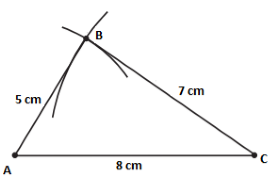Step 2: Here we can see that AC is diagonal, so D will be somewhere opposite to B with reference to AC.

AD = 6.5 cm so draw an arc from A as the centre with radius 6.5 cm.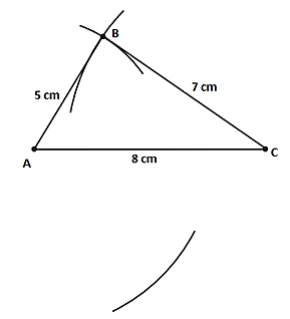Step 3: Now draw an arc with C as the centre and by taking radius 6 cm so that it intersects the above arc.Step 4: The point of intersection of the two arcs is point D. Now join AD and DC to complete the quadrilateral.

Hence, ABCD is the required quadrilateral.

2. If  two diagonals and three sides of the quadrilateral are given

Example

Construct a quadrilateral ABCD if the two diagonals are AC = 6.5 cm and BD = 8 cm. The other sides are BC = 5.5 cm, AD = 6.5 cm and CD = 6 cm.

Solution

First of all, draw a rough sketch of the quadrilateral by using the given measurements. Then start constructing the real one.

Step 1: We can see that AD, AC and DC are given so we can construct a triangle ΔACD by using SSS criterion.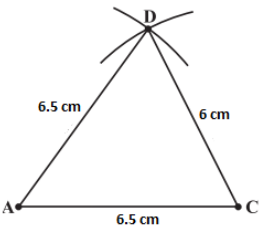Step 2: Now, we know that BD is given so we can draw the point B keeping D as the centre and draw an arc of radius 8 cm just opposite to the point D with reference to AC.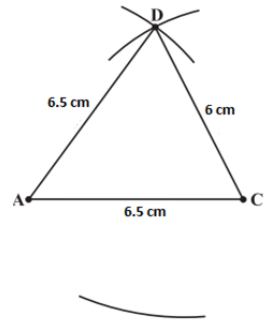Step 3: BC is given so we can draw an arc keeping C as centre and radius 5.5 cm so that it intersects the other arc.Step 4: That point of intersection of the arcs is point B. Join AB and BC to complete the quadrilateral.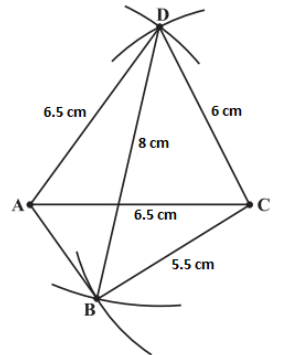3. If three angles and two adjacent sides of the quadrilateral are given.

Example

Construct a quadrilateral ABCD in which the two adjacent sides are AB = 4.5 cm and BC = 7.5 cm. The given three angles are ∠A = 75ᵒ, ∠B = 105ᵒ and ∠C = 120ᵒ.

Solution

Draw a rough sketch so that we can construct easily.

Step 1: Draw AB = 4.5 cm. Then measure ∠B = 105° using protractor and draw BC = 7.5 cm.Step 2: Draw ∠C = 120°.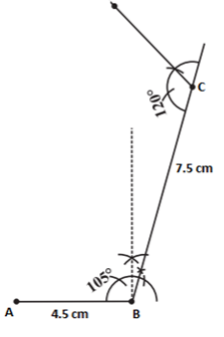Step 3: Measure ∠A = 75° and make a line until it touches the line coming from point C.4. If the three sides with two included angles of the quadrilateral are given.

Example

Construct a quadrilateral ABCD in which the three sides are AB = 5 cm, BC = 6 cm and CD = 7.5 cm. The two included angles are ∠B = 105° and ∠C = 80°.

Solution

Draw a rough sketch.

Step 1: Draw the line BC = 6 cm. Then draw ∠B = 105° and mark the length of AB = 5 cm.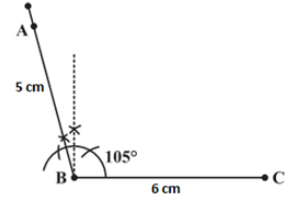Step 2: Draw ∠C = 80° using protractor towards point B.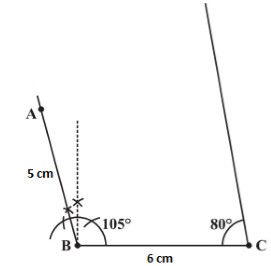Step 3: Mark the length of CD i.e.7.5 cm from C to make CD = 7.5 cm.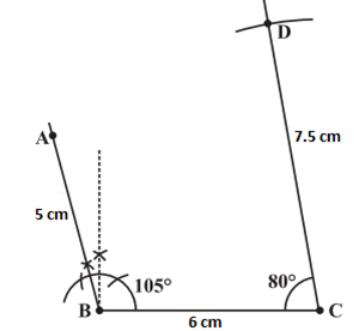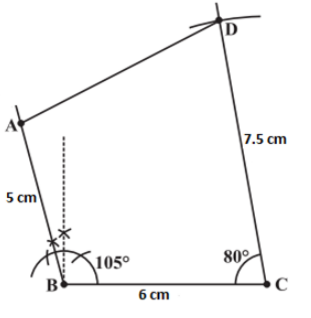Hence ABCD is the required quadrilateral.

Some Special Cases

There are some special cases in which we can construct the quadrilateral with less number of measurements also.

Example

Construct a square READ with RE = 5.1 cm.

Solution

Given Re = 5.1 cm.

As it is a special quadrilateral called square, we can get more details out of it.

a. All sides of square are equal, so RE = EA = AD = RD = 5.1 cm.

b. All the angles of a square are 90°, so ∠R = ∠E = ∠A = ∠D = 90°

Step 1: Draw a rough sketch of the square.Step 2: To construct a square, draw a line segment RE = 5.1 cm. Then draw the angle of 90° at both ends R and E of the line segment RE.Step 3: As all the sides of the square READ are equal, draw the arc of 5.1 cm from the vertex R and E to cut the lines RD and EA respectively.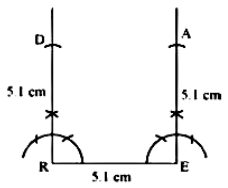Step 4: Join A and D to make a line segment AD.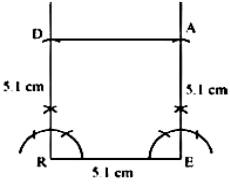```### Course Features

• 728 Video Lectures
• Revision Notes
• Previous Year Papers
• Mind Map
• Study Planner
• NCERT Solutions
• Discussion Forum
• Test paper with Video Solution